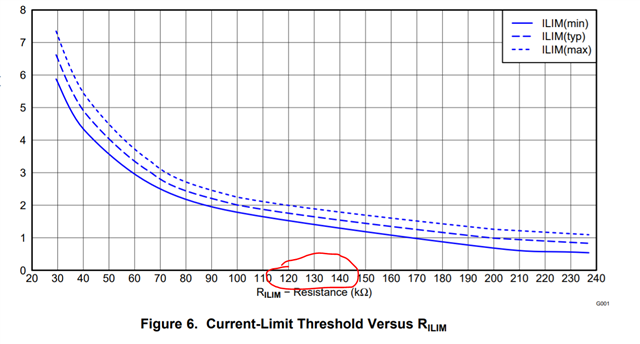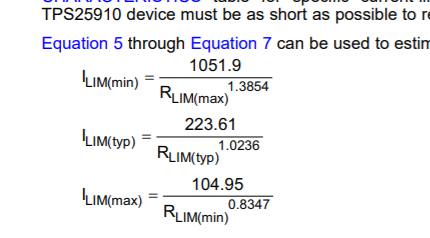If you have a related question, please click the "Ask a related question" button in the top right corner. The newly created question will be automatically linked to this question.

# TPS25910: TPS25910RSAR ILIM configuration

Part Number: TPS25910
Other Parts Discussed in Thread: TPS25947, TPS2596

Hello,

I am using TPS25910RSAR on my design. To limit the output current, I have a resistor (110K) connected to the LIM pin and GNG. My desired maximum current limit is 1.75A. Higher than this will cause heating up my DC to DC converter (TPS562200DDCR) and consequently thermal shutdown.

Our desired min current value is 1.5A and the max value is 1.75A. according to the datasheet, I think that's impossible to maintain the range! Do you have a solution to fulfill our requirements? we need to stay between 1.5 to 1.75A.

Many thanks,

Jafar

• Hi Jafar,

Based on your requirement looks you have to choose resistor between 120K-140K , but still its hard to guarantee range you want. Also you have to consider tolerance of resistor at ILIM pin. You can check better accuracy parts like TPS2596,TPS25947.Regards

Kunal Goel

• Hello Kunal,

Something is not clear for us. there are 3 different equations in the datasheet to calculate the minimum, maximum and typical currents based on Rmin, Rtyp, and Rmax. let's say, we are using an ideal resistor (tollerance=0) of 130K. if we put this value of 130K on those 3 equations, we will get different values of the current!! so, it doesn't matter how accurate our resistor is, we still have a big range of the tolerance at the output current. this is confusing.

I have calculated with a 130K (1%) resistor, here are the results: Imin=1.23A, Ityp=1.53A, and Imax=1.79A. if we go for a 0.5% resistor, these value results will not change a lot!

we want to know, for example, when the shutdown happens if we reach Imin=1.23A. in what other parameters does it depend?

As I wrote before, we need to stay in the range of 1.5A to 1.75A

Thanks,

Jafar

• Hi Jafar,

Variation in current limit depends on process ,voltage and temp.  What you can do is first put 1.5A in minimum current limit formula and calculate R  and then use that R to estimate max value of current limit.Regards

Kunal Goel